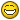# Db Scale

I have a problem that has been bugging me for some time now and I’d really like an answer for it, so if someone could help out, thanks.Here is an image of what I’m talking about:It’s a fragment of the dB scale taken from a sound file loaded into Sound Forge, and clearly shows that when converting the logarithmic dB scale to a linear one, -6 dB (or -6.02 to be exact) equals 50%. And -2.5 dB is half of that, or 25%. You can also find this out when normalising the sound file to a certain dB value in Sound Forge, as it also gives a linear percentage value alongside it.

Or here is a page with a list of dB values already converted in such a way:

Now the thing I’d like to know is, what is the formula used for converting these logarithmic values to linear ones? Specifically, if you look at the above picture, -2.5 dB is clearly half of -6.02 dB, while -4.08 dB (or 4.1) is half of -12.04 dB.

In the first case I could convert -6.02 dB to get 50%, and multiplying this by 1.5 gives 75%, or -2.5 dB. But in the second case, multiplying -12.04 dB (25%) by 1.5 gives 37.5%, or -8.52 dB. So clearly there is some sort of logarithmic scale going on that I need to convert to a linear one.

So what kind of formula would I need in order to, say, find out the dB value of half of -18.06 dB as shown in the image? It would be half way between -4.08 and -6.02 dB, but is there a formula that I can use to work this out?

EDIT: Screwed up the title. It should really have read “converting between log and linear”

dB scale is a relative scale, it describes a relation. It is just a number, nondimensional.
So in order to calculate an absolute level, you need to set a reference level.
In your case, since you don’t want a voltage level but a percentage, your reference level is 100%.

dB = 20 * log (out_level / ref_level)

example:

-6dB = 20 * log (50% / 100%)

or

50% = 100% * 10^(-6 / 20)

-6dB always halfs. No matter from where you start. Half of -18dB therefor is -24dB.

Thanks, but I’ve since found the answer long ago already. Thanks though!